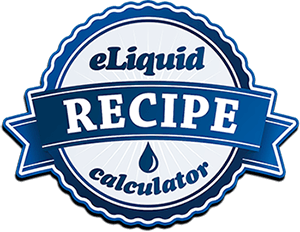# // <![CDATA[ \$('#nicBaseInput').focus(function () { \$('#mlmg1').css('color', '#1274d5'); }).blur(function() { \$('#mlmg1').css('color', '#888'); }) \$('#targetNicInput').focus(function () { \$('#mlmg2').css('color', '#1274d5'); }).blur(function() { \$('#mlmg2').css('color', '#888'); }) \$('#amountInput').focus(function () { \$('#ml1').css('color', '#1274d5'); }).blur(function() { \$('#ml1').css('color', '#888'); }) \$('#waterAddedInput').focus(function () { \$('#percent1').css('color', '#1274d5'); }).blur(function() { \$('#percent1').css('color', '#888'); }) \$('#flavorPercentInput').focus(function () { \$('#percent2').css('color', '#1274d5'); }).blur(function() { \$('#percent2').css('color', '#888'); }) //Allows ONLY Numbers and Decimal inside Input. function isNumberKey(evt) { var charCode = (evt.which) ? evt.which : evt.keyCode; if (charCode != 46 && charCode > 31 && (charCode < 48 || charCode > 57)) return false; return true; }; function Calc (a,b,c,d,e){ this.a = a; this.b = b; this.c = c; this.d = d; this.e = e; }; Calc.prototype.withNicMl = function (){ return this.b * this.c / this.a; }; Calc.prototype.waterml = function (){ return this.c/100*this.d; }; Calc.prototype.flavorml = function (){ return this.c/100*this.e; }; Calc.prototype.woNicml = function (){ return this.c-(this.withNicMl())-(this.waterml())-(this.flavorml()); }; Calc.prototype.nicDrop = function (){ return 20*this.withNicMl(); }; Calc.prototype.woNicDrop = function (){ return 20*this.woNicml(); }; Calc.prototype.waterDrop = function (){ return 20*this.waterml(); }; Calc.prototype.flavorDrop = function (){ return 20*this.flavorml(); }; Calc.prototype.nicPercent = function(){ return this.withNicMl()/this.c*100; }; Calc.prototype.woNicPercent = function (){ return this.woNicml()/this.c*100 }; Calc.prototype.waterPercent = function () { return this.waterml()/this.c*100; }; Calc.prototype.flavorPercent = function(){ return this.flavorml()/this.c*100; }; function reset (x1,x2,x3,x4,d1,d2,d3,d4,p1,p2,p3,p4){ x1 = "00.0"; x2 = "00.0"; x3 = "00.0"; x4 = "00.0"; d1 = "00.0"; d2 = "00.0"; d3 = "00.0"; d4 = "00.0"; p1 = "00.0"; p2 = "00.0"; p3 = "00.0"; p4 = "00.0"; } \$(function(){ \$("form").submit(function(event){ event.preventDefault(); var nicBaseInput = parseInt(\$("#nicBaseInput").val()); var targetNicInput = parseInt(\$("#targetNicInput").val()); var amountInput = parseInt(\$("#amountInput").val()); var waterInput = parseInt(\$("#waterAddedInput").val()); var flavor = parseInt(\$("#flavorPercentInput").val()); if ((\$("#nicBaseInput").val()==='') && (\$("#amountInput").val()==='')) { alert("Please enter Nictine Base and Amount!"); reset(newCalc.withNicMl(),newCalc.woNicml(),newCalc.waterml(),newCalc.flavorml(),newCalc.nicDrop(),newCalc.woNicDrop(),newCalc.waterDrop(),newCalc.flavorDrop(),newCalc.nicPercent(),newCalc.woNicPercent(),newCalc.waterPercent(),newCalc.flavorPercent()); } else if ((\$("#nicBaseInput").val()==='') || (\$("#amountInput").val()==='')){ alert("Please enter Nicotine Base or Amount!"); reset(newCalc.withNicMl(),newCalc.woNicml(),newCalc.waterml(),newCalc.flavorml(),newCalc.nicDrop(),newCalc.woNicDrop(),newCalc.waterDrop(),newCalc.flavorDrop(),newCalc.nicPercent(),newCalc.woNicPercent(),newCalc.waterPercent(),newCalc.flavorPercent()); } else if(\$("#waterAddedInput").val()===''){ waterInput = 0; }; var newCalc = new Calc(nicBaseInput,targetNicInput,amountInput,waterInput,flavor); \$("#nicOutputMl").text((newCalc.withNicMl()).toFixed(2)); \$("#withOutMl").text((newCalc.woNicml()).toFixed(2)); \$("#waterMl").text((newCalc.waterml()).toFixed(2)); \$("#flavorml").text((newCalc.flavorml()).toFixed(2)); \$("#nicDrop").text((newCalc.nicDrop()).toFixed(2)); \$("#woNicDrop").text((newCalc.woNicDrop()).toFixed(2)); \$("#waterDrop").text((newCalc.waterDrop()).toFixed(2)); \$("#flavorDrop").text((newCalc.flavorDrop()).toFixed(2)); \$("#nicPercent").text((newCalc.nicPercent()).toFixed(2)); \$("#woNicPercent").text((newCalc.woNicPercent()).toFixed(2)); \$("#waterPercent").text((newCalc.waterPercent()).toFixed(2)); \$("#flavorPercent").text((newCalc.flavorPercent()).toFixed(2)); }); }); // ]]>Fill out our intuitive form above to calculate your desired eLiquid Recipes.

PG or VG with Nicotine
PG or VG w/out Nicotine
Water
FlavoringDrops are an estimation. 20 drops of water from a dropper equals about 1ml.